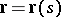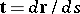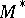# Affine pseudo-distance

The number, equal to the modulus of the vector product of the vectorsand, whereis an arbitrary point in an equi-affine plane,is a point on a plane curve,is the affine parameter of the curve andis the tangent vector at the point. This numberis called the affine pseudo-distance fromto. Ifis held fixed, whileis moved along the curve, the affine pseudo-distance fromtowill assume a stationary value if and only iflies on the affine normal of the curve at. An affine pseudo-distance in an equi-affine space can be defined in a similar manner for a given hypersurface.